Select Page

# CBSE MCQ Maths 12 Science Relations and Functions Answers in English

CBSE MCQ Maths 12 Science Relations and Functions Answers in English to enable students to get Answers in a narrative video format for the specific question.

Expert Teacher provides CBSE MCQ Maths 12 Science Relations and Functions Answers through Video Answers in English language. This video solution will be useful for students to understand how to write an answer in exam in order to score more marks. This teacher uses a narrative style for a question from Relations and Functions not only to explain the proper method of answering question, but deriving right answer too.

Please find the question below and view the Answer in a narrative video format.

Question:

## Similar Questions from CBSE, 12th Science, Maths, Relations and Functions

Question 1 : Number of binary sets on the set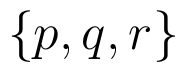is, (View Answer Video)

Question 2 : Let * be the binary operation on N given by a * b = LCM of a and b. Find 20 * 16. (View Answer Video)

Question 3 : A function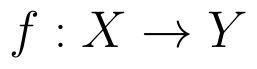is bijective if and only if, (View Answer Video)

Question 5 : Let A = {1, 2, 3, 4} and let R = {(2, 2), (3, 3), (4, 4), (1, 2)} be a relation on A. Then, R is, (View Answer Video)

### Application of Derivatives

Question 1 : A cylindrical tank of radius 10 m is being filled with wheat at the rate of the 314 cubic meters per hour. Then the depth of the wheat is increasing at the rate of ___________. (View Answer Video)

Question 2 : Find two numbers whose sum is 24 and whose product is as large as possible. (View Answer Video)

Question 3 : The rate of change of the area of a circle with respect to its radius r at r=6cm is: (View Answer Video)

Question 4 : A tank with a rectangular base and rectangular sides, open at the top is to be constructed so that its depth is 2m and volume is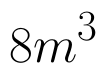If the building of tank costs Rs.70 per sq meter for the base and Rs.45 per sq meter for sides. What is the cost of least expensive? (View Answer Video)

Question 5 : The point on the curve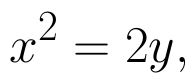which is nearest to the point (0, 5) is :

### Integrals

Question 1 : Evaluate :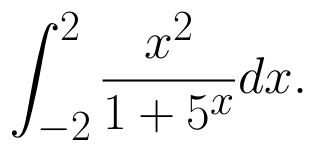(View Answer Video)

Question 2 : Find :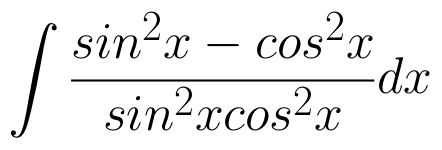(View Answer Video)

Question 3 : Evaluate :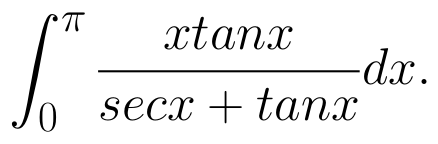(View Answer Video)

Question 4 : Find :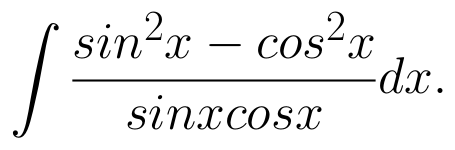(View Answer Video)

Question 5 : Evaluate :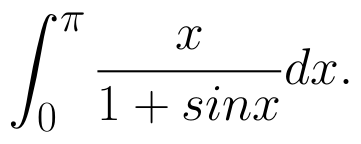(View Answer Video)

### Linear Programming

Question 1 : The objective function is maximum or minimum, which lies on the boundary of the feasible region. (View Answer Video)